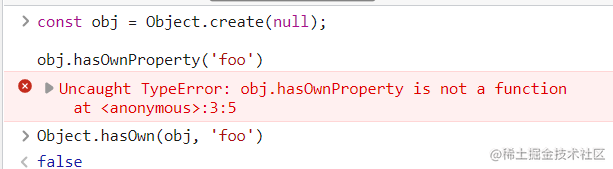2022-09-1800

# ES6 对象的新增方法

## 1. `Object.is()` ——只要两个值是一样的，它们就应该相等

ES5 比较两个值是否相等，只有两个运算符：相等运算符（ `==`）和严格相等运算符（ `===`）。它们都有缺点，前者会自动转换数据类型，后者的 `NaN`不等于自身，以及 `+0`等于 `-0`。JavaScript 缺乏一种运算，在所有环境中， 只要两个值是一样的，它们就应该相等

ES6 提出" Same-value equality"（同值相等）算法，用来解决这个问题。 `Object.is`就是部署这个算法的新方法。它用来比较两个值是否严格相等， 与严格比较运算符（===）的行为基本一致

``````Object.is('foo', 'foo')

Object.is({}, {})``````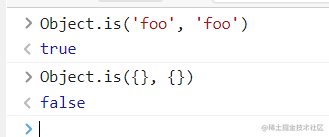``````+0 === -0 //true
NaN === NaN // false

Object.is(+0, -0) // false
Object.is(NaN, NaN) // true``````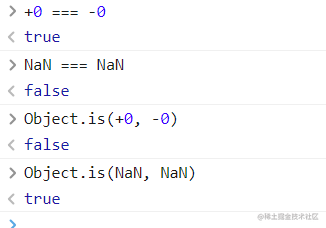ES5 可以通过下面的代码，部署 `Object.is`

``````Object.defineProperty(Object, 'is', {
value: function(x, y) {
if (x === y) {

return x !== 0 || 1 / x === 1 / y;
}

return x !== x && y !== y;
},
configurable: true,
enumerable: false,
writable: true
});``````

## 2. `Object.assign()` ——用于多个对象的合并

### 2.1 基本用法

`Object.assign()`方法用于对象的合并，将源对象（source）的所有可枚举属性，复制到目标对象（target）。

``````const target = { a: 1 }

const source1 = { b: 2 }
const source2 = { c: 3 }

Object.assign(target, source1, source2)
target // {a:1, b:2, c:3}``````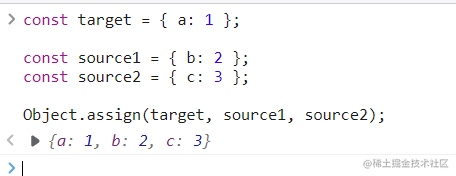** `Object.assign()` 方法的第一个参数是目标对象，后面的参数都是源对象。**

``````const target = { a: 1, b: 1 }

const source1 = { b: 2, c: 2 }
const source2 = { c: 3 }

Object.assign(target, source1, source2)
target // {a:1, b:2, c:3}``````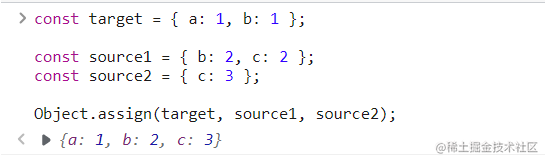#### 2.1.1 如果 `Object.assign()` 只有一个参数

``````const obj = {a: 1};
Object.assign(obj) === obj // true``````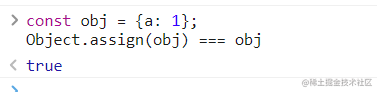``typeof Object.assign(2)``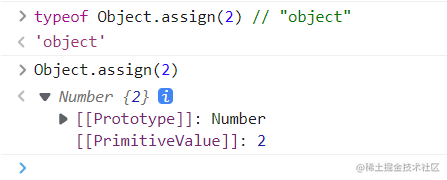``````Object.assign(undefined)
Object.assign(null)``````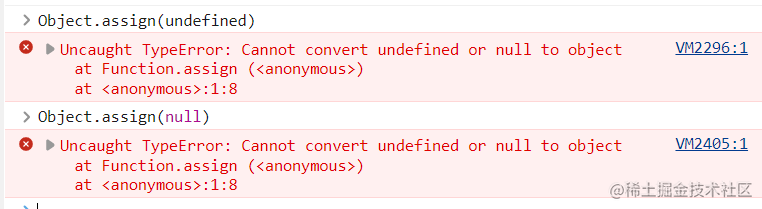#### 2.1.2 非对象参数出现在源对象的位置（即非首参数)

``````let obj = {a: 1};
Object.assign(obj, undefined) === obj
Object.assign(obj, null) === obj``````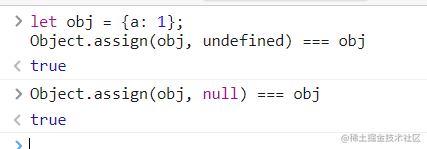``````const v1 = 'abc'
const v2 = true
const v3 = 10

const obj = Object.assign({}, v1, v2, v3)
console.log(obj)``````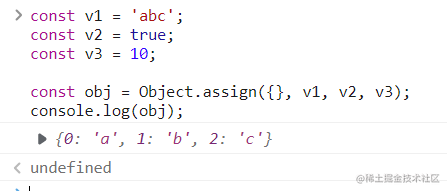#### 2.1.3 包装对象 `Numer``Boolean``String`

``````Object(true) // {[[PrimitiveValue]]: true}
Object(10)  //  {[[PrimitiveValue]]: 10}
Object('abc') // {0: "a", 1: "b", 2: "c", length: 3, [[PrimitiveValue]]: "abc"}``````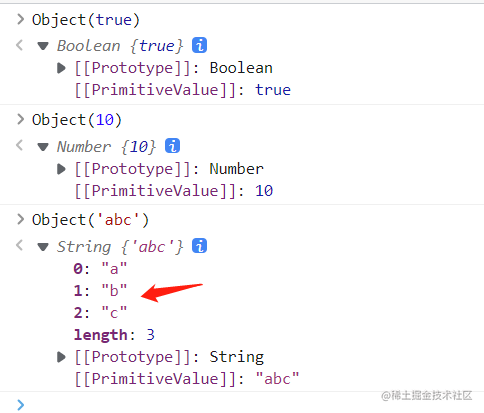#### 2.1.4 `Object.assign()` 只拷贝源对象的自身属性

`Object.assign()`拷贝的属性是有限制的， 只拷贝源对象的自身属性（不拷贝继承属性），也不拷贝不可枚举的属性（ `enumerable: false`）。

``````Object.assign({b: 'c'},
Object.defineProperty({}, 'invisible', {
enumerable: false,
value: 'hello'
})
)
// { b: 'c' }``````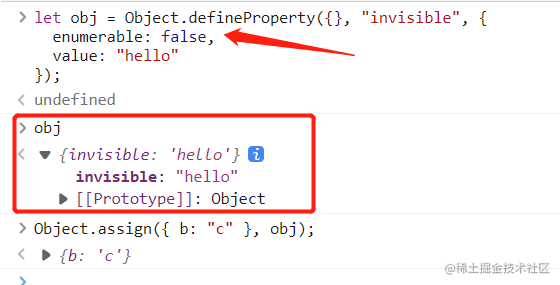``````Object.assign({ a: 'b' }, { [Symbol('c')]: 'd' })
// { a: 'b', Symbol(c): 'd' }``````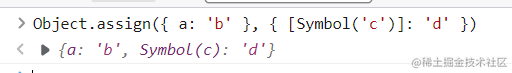### 2.2 注意点

#### （2.2.1） `Object.assign()` 浅拷贝

`Object.assign()`方法实行的是浅拷贝，而不是深拷贝。也就是说，如果源对象某个属性的值是对象，那么目标对象拷贝得到的是这个对象的引用。

``````const obj1 = {a: {b: 1}}
const obj2 = Object.assign({}, obj1)

obj1.a.b = 2
obj2.a.b // 2``````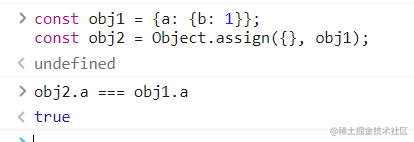#### （2.2.2）替换同名属性

``````const target = { a: { b: 'c', d: 'e' } }
const source = { a: { b: 'hello' } }
Object.assign(target, source)
// { a: { b: 'hello' } }``````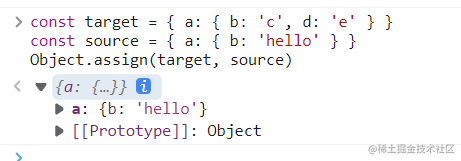#### （2.2.3）处理数组时会把数组视为对象

`Object.assign()`可以用来处理数组，但是会把数组视为对象。

``````Object.assign([1, 2, 3], [4, 5])
// [4, 5, 3]``````#### （2.2.4）取值函数求值后再复制

`Object.assign()`只能进行值的复制，如果要复制的值是一个取值函数，那么将求值后再复制。

``````const source = {
get foo() { return 1 }
};
const target = {};

Object.assign(target, source)``````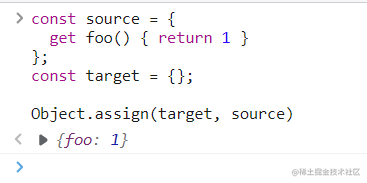### 2.3 常见用途

`Object.assign()`方法有很多用处。

（1）为对象添加属性

``````class Point {
constructor(x, y) {
Object.assign(this, {x, y});
}
}``````

（2）为对象添加方法

``````Object.assign(SomeClass.prototype, {
someMethod(arg1, arg2) {
···
},
anotherMethod() {
···
}
});

SomeClass.prototype.someMethod = function (arg1, arg2) {
···
};
SomeClass.prototype.anotherMethod = function () {
···
};``````

（3）克隆对象

``````function clone(origin) {
return Object.assign({}, origin);
}``````

``````function clone(origin) {
let originProto = Object.getPrototypeOf(origin);
return Object.assign(Object.create(originProto), origin);
}``````

（4）合并多个对象

``````const merge =
(target, ...sources) => Object.assign(target, ...sources)``````

``````const merge =
(...sources) => Object.assign({}, ...sources)``````

（5）为属性指定默认值

``````const DEFAULTS = {
logLevel: 0,
outputFormat: 'html'
}

function processContent(options) {
options = Object.assign({}, DEFAULTS, options)
console.log(options)
// ...

}``````

``````const DEFAULTS = {
url: {
host: 'example.com',
port: 7070
},
};

processContent({ url: {port: 8000} })
// {
//   url: {port: 8000}
// }``````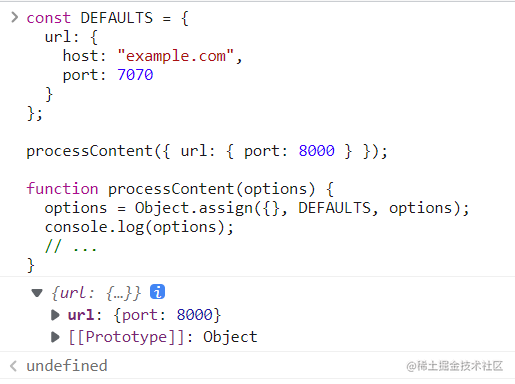## 3. Object.getOwnPropertyDescriptors()——返回对象所有属性的描述对象

ES5 的 `Object.getOwnPropertyDescriptor()`方法会返回某个对象属性的描述对象（descriptor）。ES2017 引入了 `Object.getOwnPropertyDescriptors()`方法，返回指定对象所有自身属性（非继承属性）的描述对象。

``````const obj = {
foo: 123,
get bar() { return 'abc' }
};

Object.getOwnPropertyDescriptors(obj)
// { foo:
//    { value: 123,
//      writable: true,
//      enumerable: true,
//      configurable: true },
//   bar:
//    { get: [Function: get bar],
//      set: undefined,
//      enumerable: true,
//      configurable: true } }``````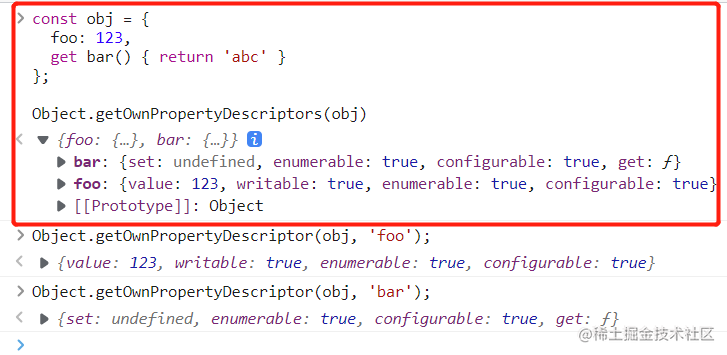``````function getOwnPropertyDescriptors(obj) {
const result = {};
for (let key of Reflect.ownKeys(obj)) {
result[key] = Object.getOwnPropertyDescriptor(obj, key);
}
return result;
}

### 3.1 正确拷贝 `get` 属性和 `set` 属性

``````const source = {
set foo(value) {
console.log(value);
}
};

const target1 = {};
Object.assign(target1, source);

Object.getOwnPropertyDescriptor(target1, 'foo')``````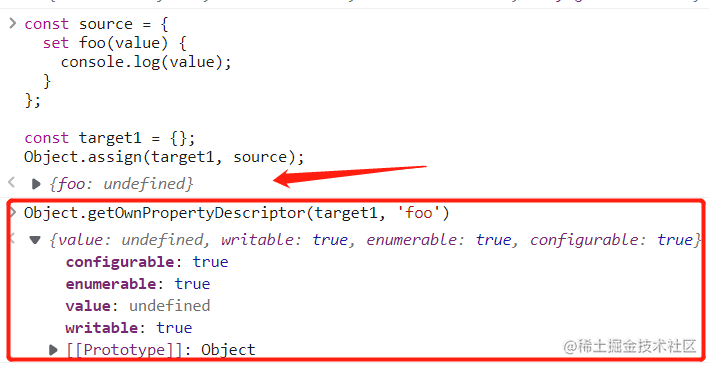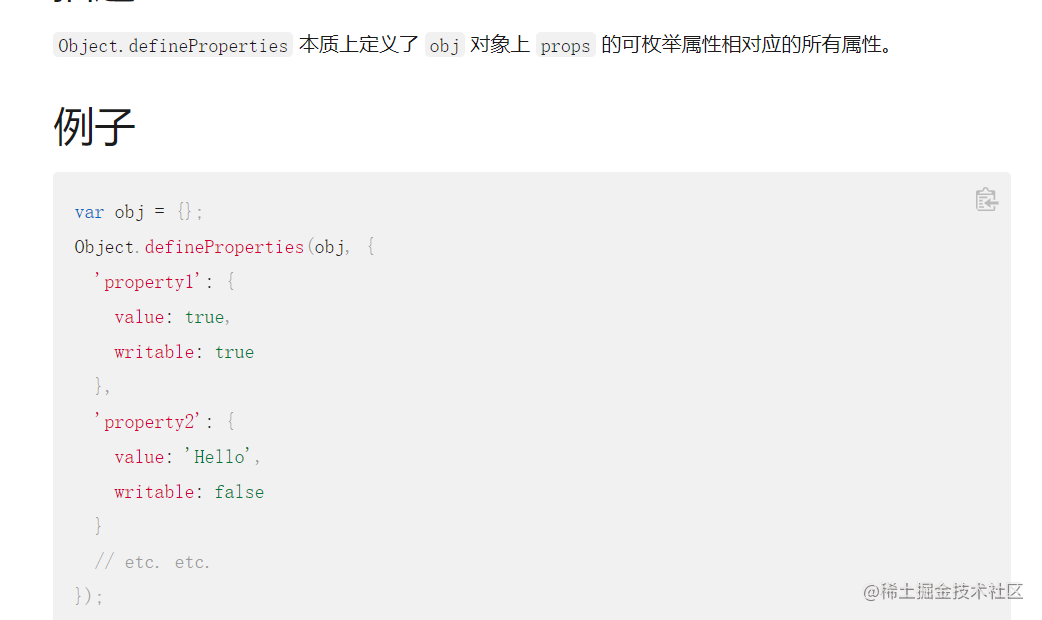``````const source = {
set foo(value) {
console.log(value);
}
};

const target2 = {};
Object.defineProperties(target2, Object.getOwnPropertyDescriptors(source));
Object.getOwnPropertyDescriptor(target2, 'foo')``````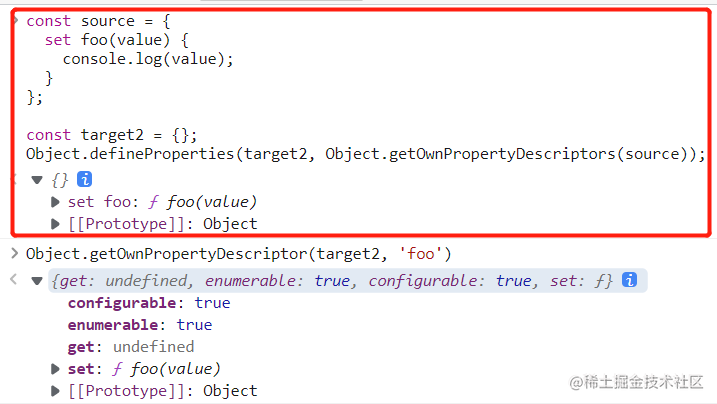``````const shallowMerge = (target, source) => Object.defineProperties(
target,
Object.getOwnPropertyDescriptors(source)
);

### 3.2 配合 `Object.create()` 克隆对象属性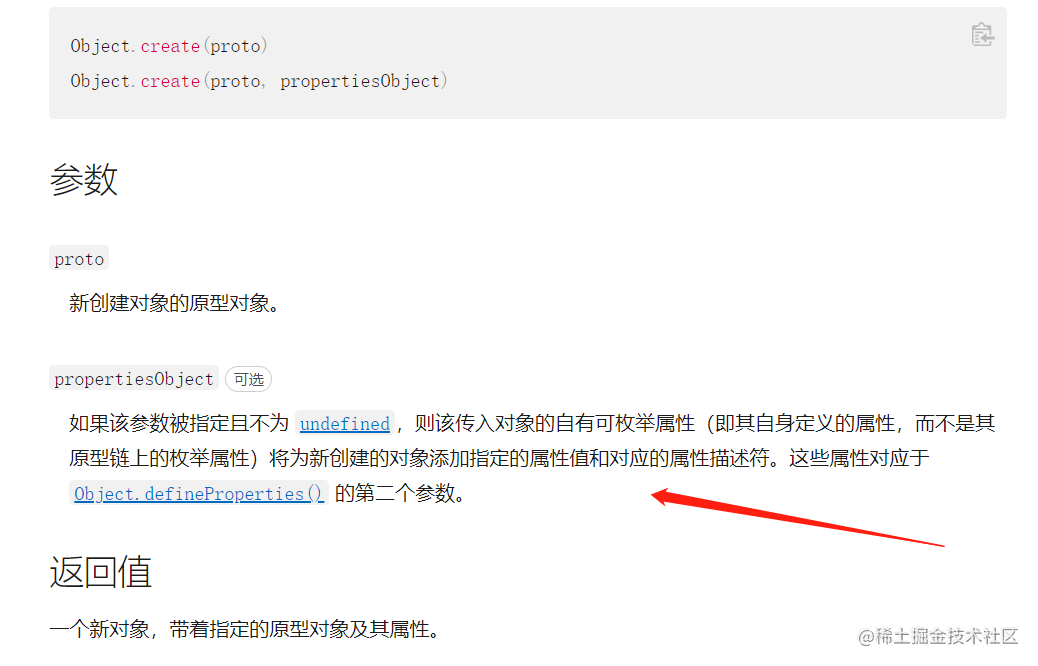`Object.getOwnPropertyDescriptors()`方法的另一个用处，是配合 `Object.create()`方法，将对象属性克隆到一个新对象。这属于浅拷贝。

``````const clone = Object.create(Object.getPrototypeOf(obj),
Object.getOwnPropertyDescriptors(obj));

const shallowClone = (obj) => Object.create(
Object.getPrototypeOf(obj),
Object.getOwnPropertyDescriptors(obj)
);``````

### 3.3 实现一个对象继承另一个对象

``````const obj = {
__proto__: prot,
foo: 123,
}``````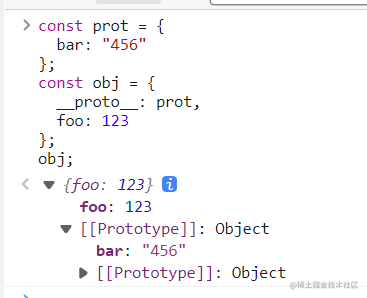ES6 规定 `__proto__` 只有浏览器要部署，其他环境不用部署。如果去除 `__proto__`，上面代码就要改成下面这样。

``````const obj = Object.create(prot)
obj.foo = 123

// 或者

const obj = Object.assign(
Object.create(prot),
{
foo: 123,
}
)``````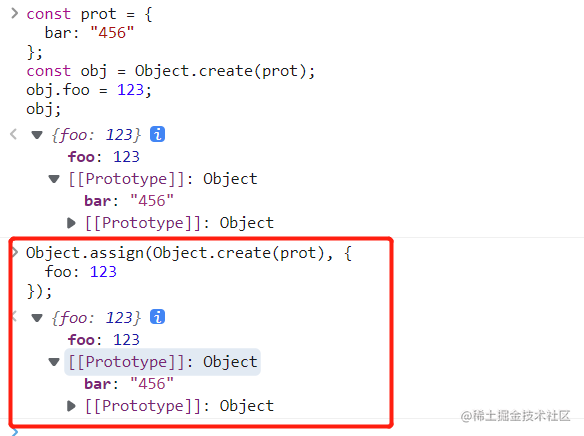``````const obj = Object.create(
prot,
Object.getOwnPropertyDescriptors({
foo: 123,
})
);``````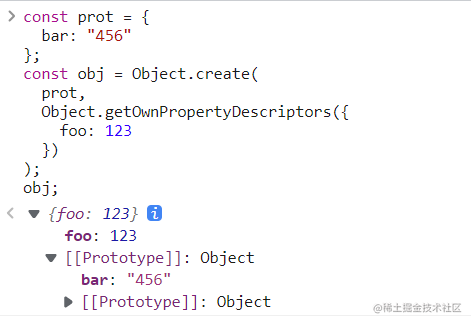`Object.getOwnPropertyDescriptors()`也可以用来实现 Mixin（混入）模式。

``````let mix = (object) => ({
with: (...mixins) => mixins.reduce(
(c, mixin) => Object.create(
c, Object.getOwnPropertyDescriptors(mixin)
), object)
});

let a = {a: 'a'};
let b = {b: 'b'};
let c = {c: 'c'};
let d = mix(c).with(a, b);

d.c
d.b
d.a``````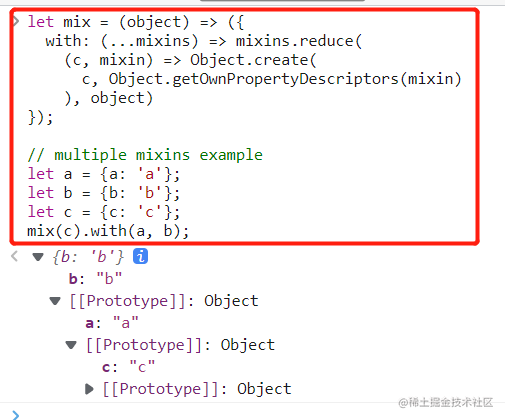## 4. `__proto__` 属性， `Object.setPrototypeOf()` ，Object.getPrototypeOf()

JavaScript 语言的对象继承是通过原型链实现的。ES6 提供了更多原型对象的操作方法。

### 4.1 `__proto__` 属性——读取或设置当前对象的原型对象

`__proto__`属性（前后各两个下划线）， 用来读取或设置当前对象的原型对象（prototype）。目前，所有浏览器（包括 IE11）都部署了这个属性。

``````// es5 的写法
const obj = {
method: function() { ... }
}
obj.__proto__ = someOtherObj

// es6 的写法
var obj = Object.create(someOtherObj)
obj.method = function() { ... }``````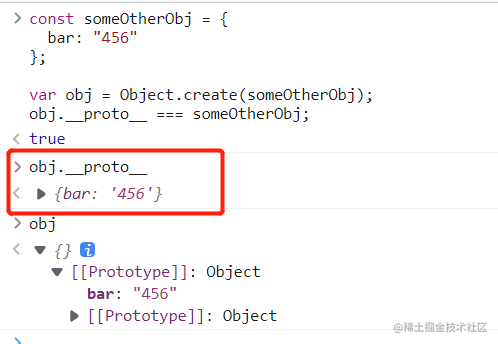``````Object.defineProperty(Object.prototype, '__proto__', {
get() {
let _thisObj = Object(this);
return Object.getPrototypeOf(_thisObj);
},
set(proto) {
if (this === undefined || this === null) {
throw new TypeError();
}
if (!isObject(this)) {
return undefined;
}
if (!isObject(proto)) {
return undefined;
}
let status = Reflect.setPrototypeOf(this, proto);
if (!status) {
throw new TypeError();
}
},
});

function isObject(value) {
return Object(value) === value;
}``````

``Object.getPrototypeOf({ __proto__: null })``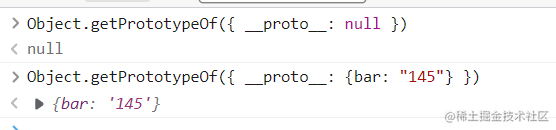### 4.2 `Object.setPrototypeOf()` ——设置一个对象的原型对象

`Object.setPrototypeOf`方法的作用与 `__proto__`相同，用来设置一个对象的原型对象（prototype）， 返回参数对象本身。它是 ES6 正式推荐的设置原型对象的方法。

``````
Object.setPrototypeOf(object, prototype)

const o = Object.setPrototypeOf({}, null);``````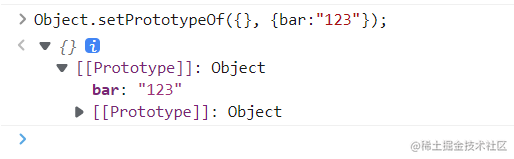``````function setPrototypeOf(obj, proto) {
obj.__proto__ = proto
return obj
}``````

``````let proto = {}
let obj = { x: 10 }
Object.setPrototypeOf(obj, proto)

proto.y = 20
proto.z = 40

obj.x // 10
obj.y // 20
obj.z // 40``````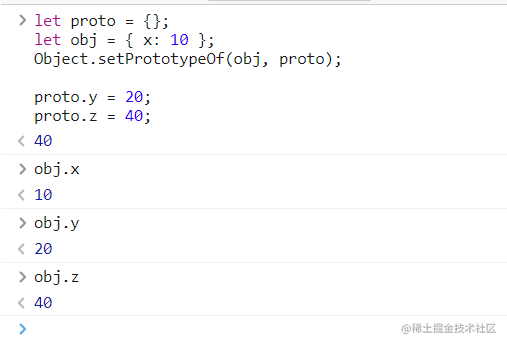``````Object.setPrototypeOf(1, {}) === 1
Object.setPrototypeOf('foo', {}) === 'foo'
Object.setPrototypeOf(true, {}) === true``````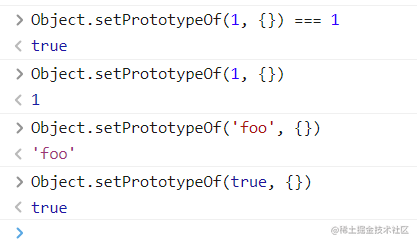``````Object.setPrototypeOf(undefined, {})

Object.setPrototypeOf(null, {})``````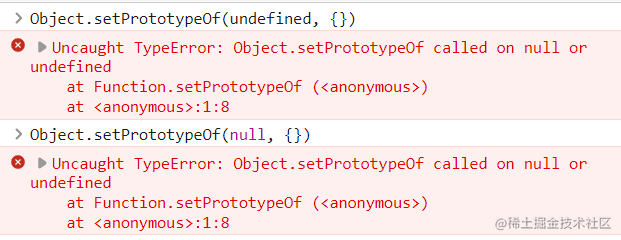### 4.3 `Object.getPrototypeOf()` ——读取一个对象的原型对象

``Object.getPrototypeOf(obj);``

``````function Rectangle() {

}

const rec = new Rectangle();

Object.getPrototypeOf(rec) === Rectangle.prototype

Object.setPrototypeOf(rec, Object.prototype);
Object.getPrototypeOf(rec) === Rectangle.prototype``````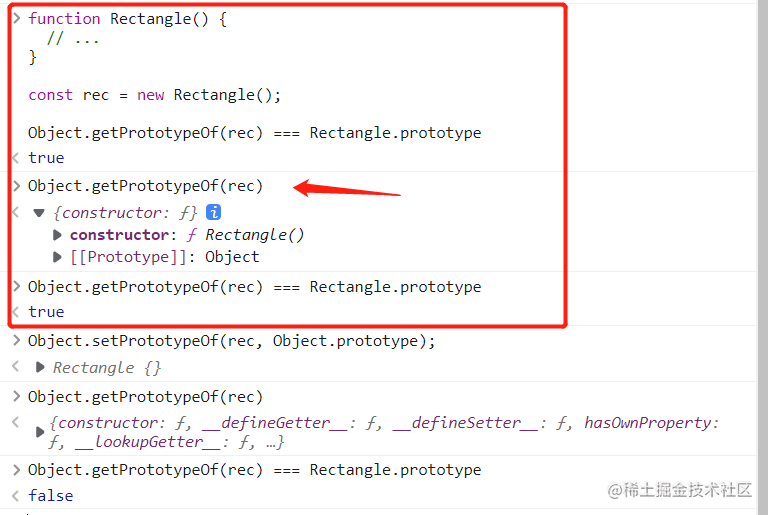``````// 等同于 Object.getPrototypeOf(Number(1))
Object.getPrototypeOf(1)
// Number {[[PrimitiveValue]]: 0}

// 等同于 Object.getPrototypeOf(String('foo'))
Object.getPrototypeOf('foo')
// String {length: 0, [[PrimitiveValue]]: ""}

// 等同于 Object.getPrototypeOf(Boolean(true))
Object.getPrototypeOf(true)
// Boolean {[[PrimitiveValue]]: false}

Object.getPrototypeOf(1) === Number.prototype // true
Object.getPrototypeOf('foo') === String.prototype // true
Object.getPrototypeOf(true) === Boolean.prototype // true``````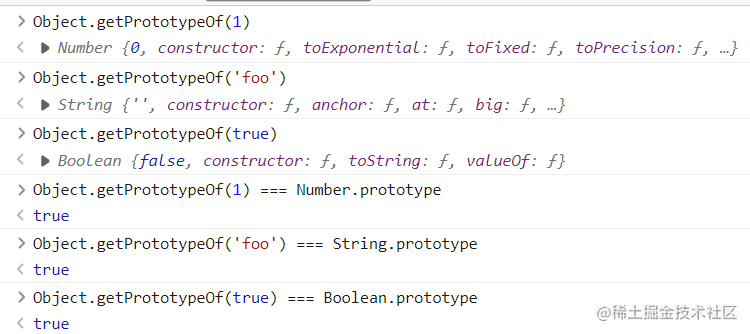``````Object.getPrototypeOf(null)

Object.getPrototypeOf(undefined)``````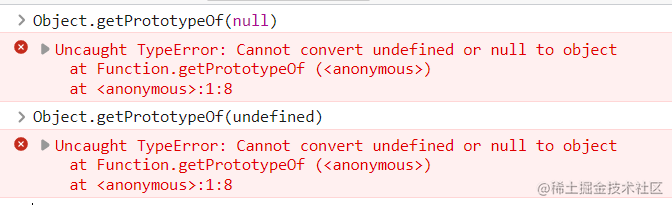## 5. Object.keys()，Object.values()，Object.entries()

### 5.1 `Object.keys() ` ——返回对象属性键名的数组

ES5 引入了 `Object.keys`方法，返回一个数组，成员是参数对象自身的（不含继承的）所有可遍历（enumerable）属性的键名。

``````var obj = { foo: 'bar', baz: 42 };
Object.keys(obj)
// ["foo", "baz"]``````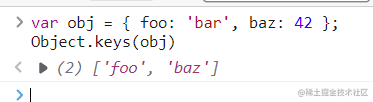ES2017 引入了跟 `Object.keys`配套的 `Object.values``Object.entries`，作为遍历一个对象的补充手段，供 `for...of`循环使用。

``````let {keys, values, entries} = Object;
let obj = { a: 1, b: 2, c: 3 };

for (let key of keys(obj)) {
console.log(key); // 'a', 'b', 'c'
}

for (let value of values(obj)) {
console.log(value); // 1, 2, 3
}

for (let [key, value] of entries(obj)) {
console.log([key, value]); // ['a', 1], ['b', 2], ['c', 3]
}``````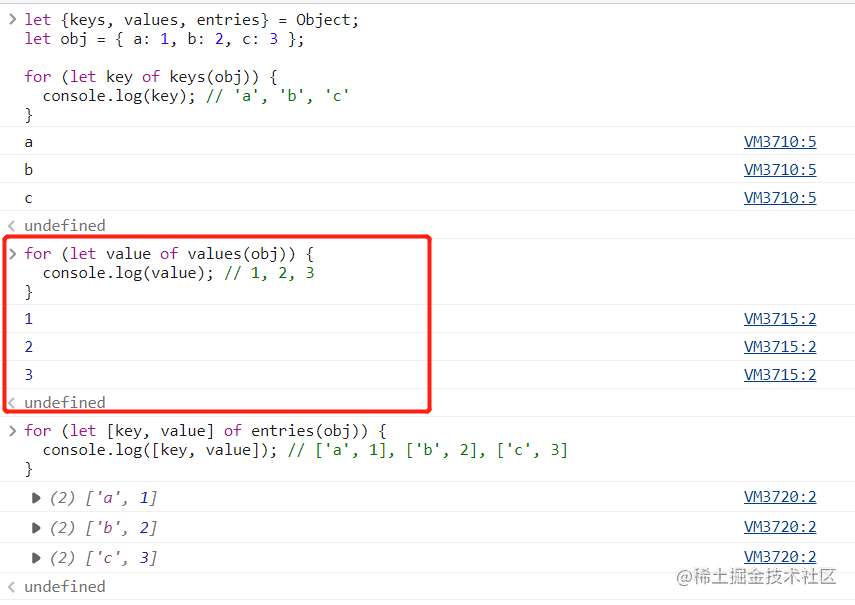### 5.2 Object.values()——返回包含对象属性键值的数组

`Object.values`方法返回一个数组，成员是参数对象自身的（不含继承的）所有可遍历（enumerable）属性的键值。

``````const obj = { foo: 'bar', baz: 42 };
Object.values(obj)
// ["bar", 42]``````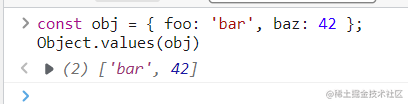``````const obj = { 100: 'a', 2: 'b', 7: 'c' };
Object.values(obj)
// ["b", "c", "a"]``````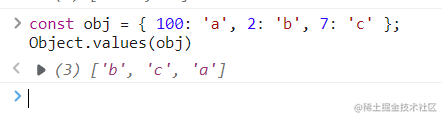#### 5.2.1 只返回对象自身的可遍历属性

`Object.values`只返回对象自身的可遍历属性。

``````const obj = Object.create({}, {p: {value: 42}});
Object.values(obj) // []``````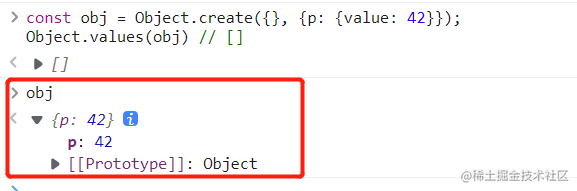``````const obj = Object.create({}, {p:
{
value: 42,
enumerable: true
}
});
Object.values(obj) // ``````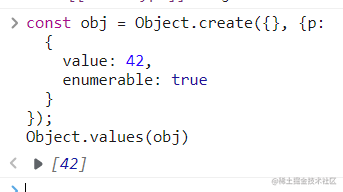#### 5.2.2 过滤属性名为 Symbol 值的属性

`Object.values`会过滤属性名为 Symbol 值的属性。

``````Object.values({ [Symbol()]: 123, foo: 'abc' });
// ['abc']``````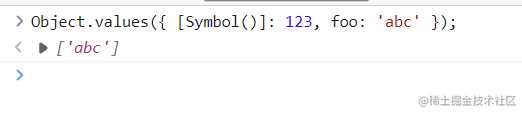``````Object.values('foo')
// ['f', 'o', 'o']``````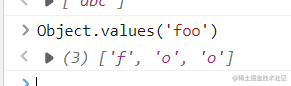``````Object.values(42)
Object.values(true)``````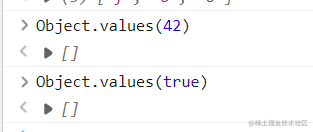### 5.3 `Object.entries()` ——返回对象属性的键值对数组

`Object.entries()`方法返回一个数组，成员是参数对象自身的（不含继承的）所有可遍历（enumerable）属性的键值对数组。

``````const obj = { foo: 'bar', baz: 42 };
Object.entries(obj)
// [ ["foo", "bar"], ["baz", 42] ]``````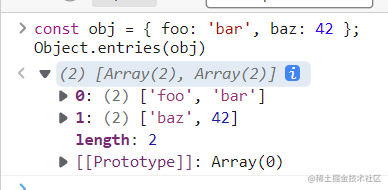``````Object.entries({ [Symbol()]: 123, foo: 'abc' });
// [ [ 'foo', 'abc' ] ]``````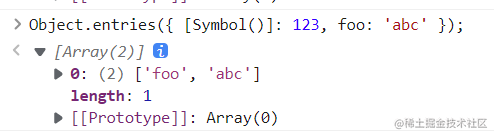#### 5.3.1 遍历对象的属性

`Object.entries`的基本用途是遍历对象的属性。

``````let obj = { one: 1, two: 2 };
for (let [k, v] of Object.entries(obj)) {
console.log(
`\${JSON.stringify(k)}: \${JSON.stringify(v)}`
);
}``````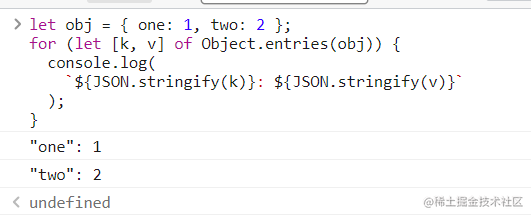#### 5.3.2 将对象转为真正的 `Map` 结构

`Object.entries`方法的另一个用处是，将对象转为真正的 `Map`结构。

``````const obj = { foo: 'bar', baz: 42 };
const map = new Map(Object.entries(obj));
map``````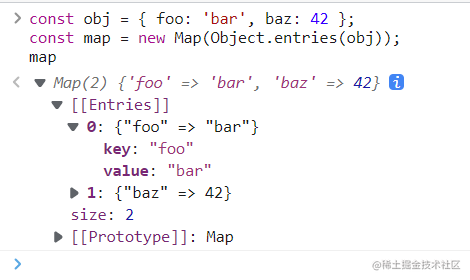``````// Generator函数的版本
function* entries(obj) {
for (let key of Object.keys(obj)) {
yield [key, obj[key]];
}
}

// 非Generator函数的版本
function entries(obj) {
let arr = [];
for (let key of Object.keys(obj)) {
arr.push([key, obj[key]]);
}
return arr;
}``````

## 6. `Object.fromEntries()` ——将一个键值对数组转为对象

`Object.fromEntries()` 方法是 `Object.entries()` 的逆操作，用于将一个键值对数组转为对象。

``````Object.fromEntries([  ['foo', 'bar'],
['baz', 42]
])
// { foo: "bar", baz: 42 }``````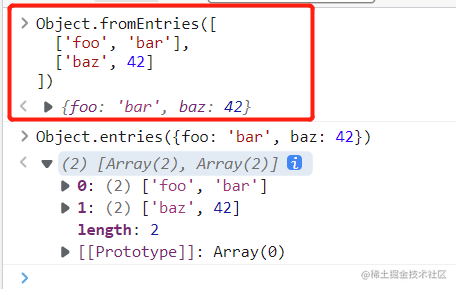#### 6.1 将 Map 结构转为对象

``````
const entries = new Map([
['foo', 'bar'],
['baz', 42]
]);

Object.fromEntries(entries)

const map = new Map().set('foo', true).set('bar', false);
Object.fromEntries(map)``````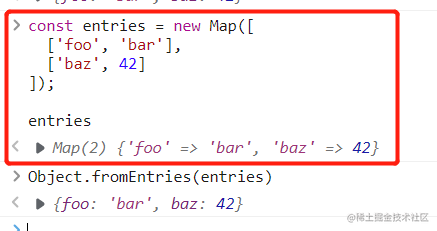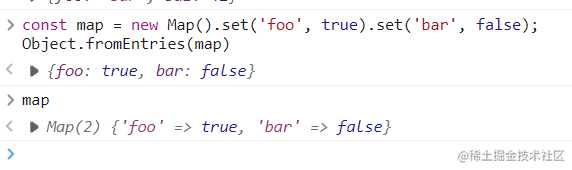``````Object.fromEntries(new URLSearchParams(
// { foo: "bar", baz: "qux" }``````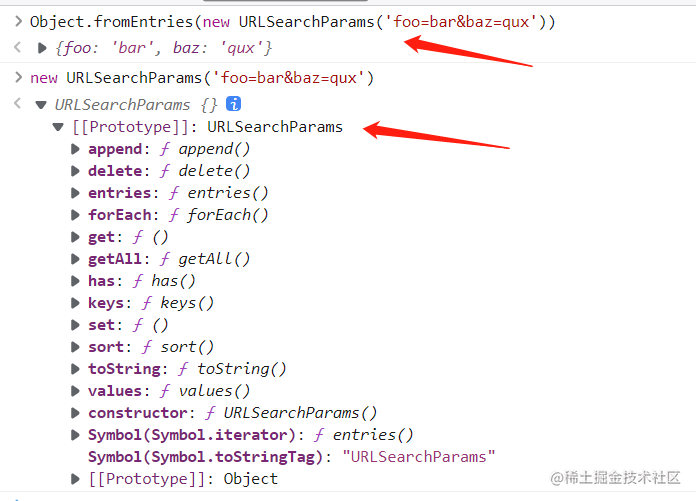## 7. `Object.hasOwn()` ——判断是否为自身的属性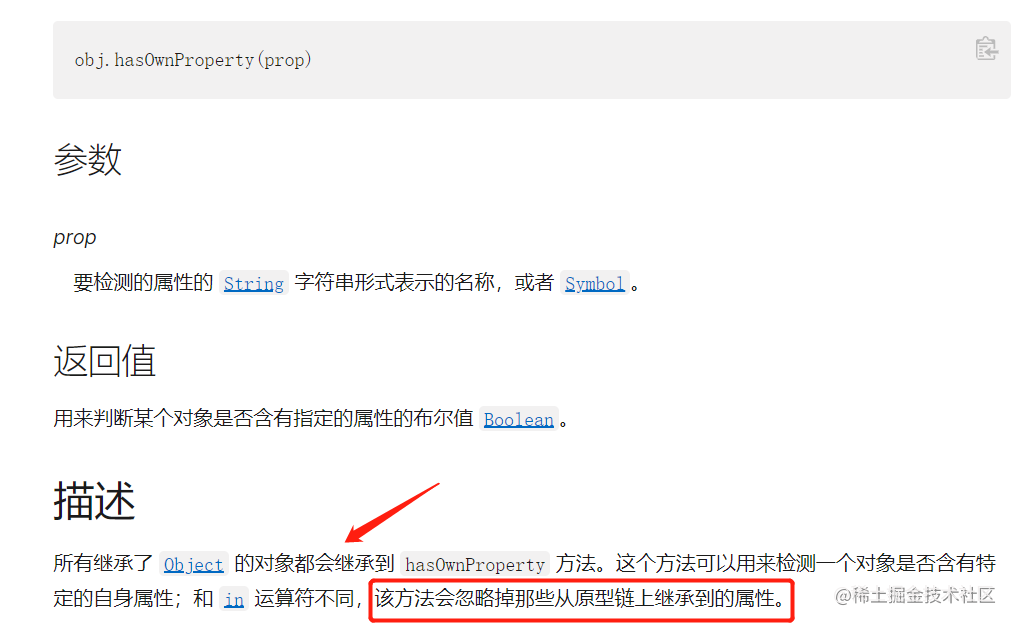JavaScript 对象的属性分成两种：自身的属性和继承的属性。对象实例有一个 `hasOwnProperty()`方法，可以判断某个属性是否为原生属性。ES2022 在 `Object`对象上面新增了一个静态方法`Object.hasOwn()`，也可以判断是否为自身的属性。

`Object.hasOwn()`可以接受两个参数，第一个是所要判断的对象，第二个是属性名。

``````const foo = Object.create({ a: 123 });
foo.b = 456;

Object.hasOwn(foo, 'a')
Object.hasOwn(foo, 'b')``````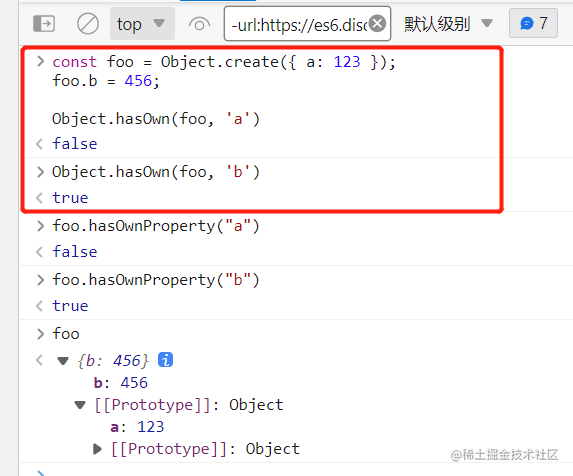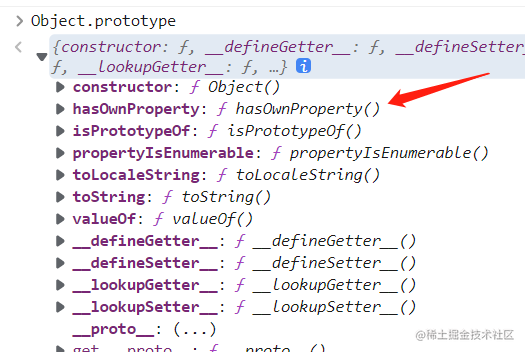`Object.hasOwn()`的一个好处是，对于不继承 `Object.prototype`的对象不会报错，而 `hasOwnProperty()`是会报错的。

``````const obj = Object.create(null);

obj.hasOwnProperty('foo')
Object.hasOwn(obj, 'foo')``````